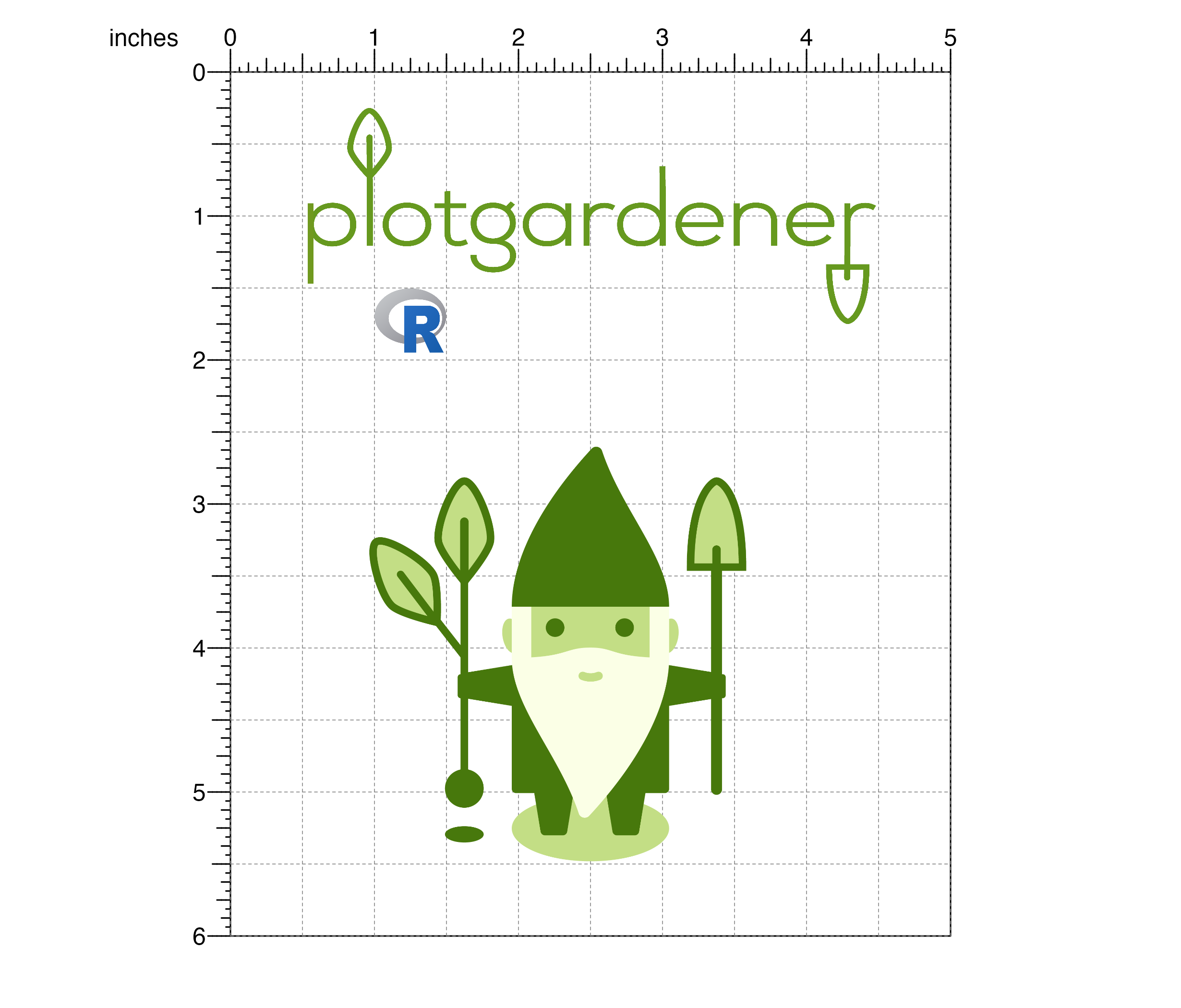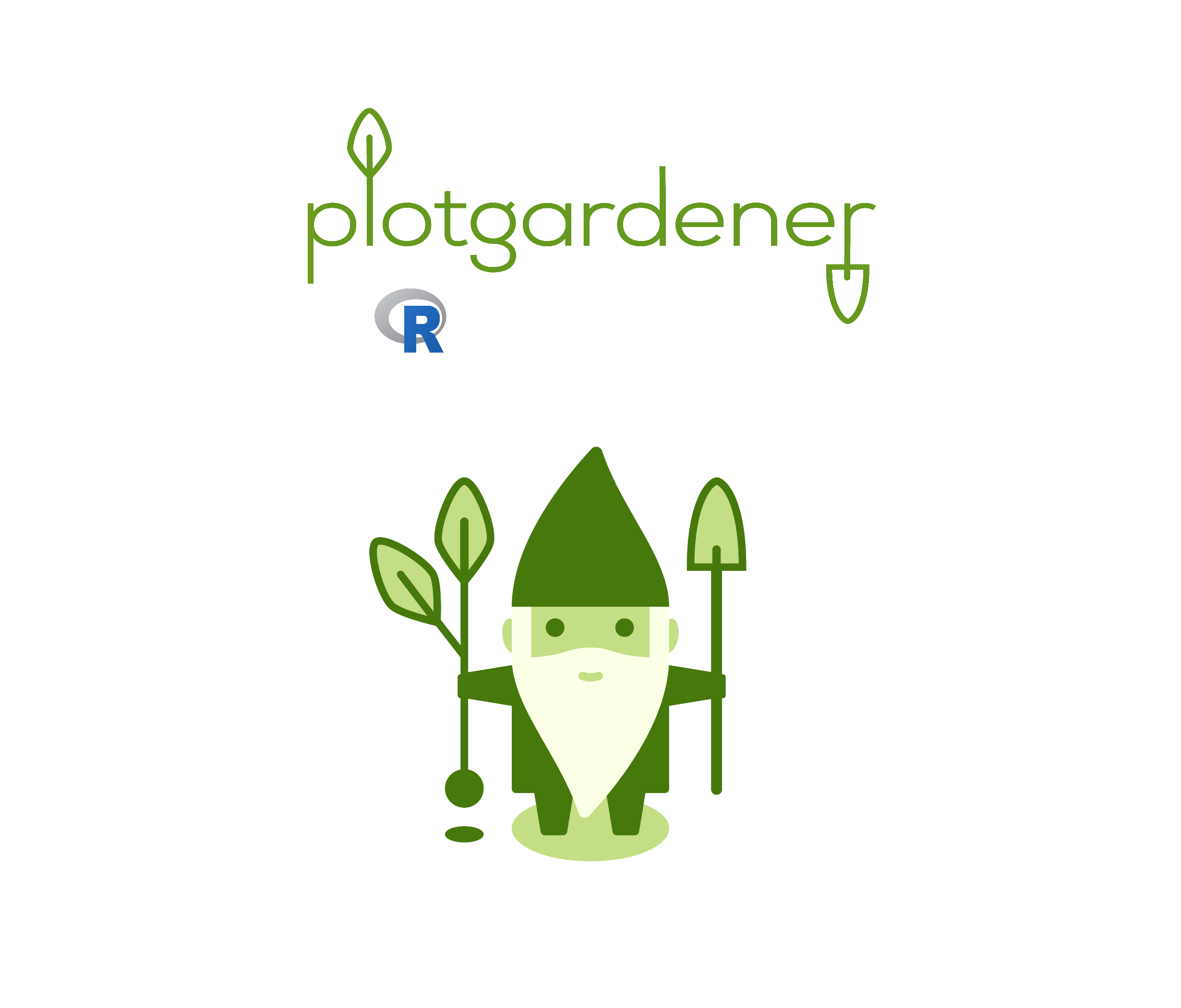Plot a raster object within a plotgardener layout

plotRaster(
image,
x,
y,
width,
height,
just = "center",
default.units = "inches",
interpolate = TRUE,
params = NULL,
...
)

## Arguments

image

Any R object that can be coerced to a raster object.

x

A numeric vector or unit object specifying raster x-locations.

y

A numeric vector, unit object, or a character vector of values containing a "b" combined with a numeric value specifying raster y-locations. The character vector will place raster y relative to the bottom of the most recently plotted plot according to the units of the plotgardener page.

width

A numeric vector or unit object specifying raster widths.

height

A numeric vector or unit object specifying raster heights.

just

Justification of text relative to its (x, y) location. If there are two values, the first value specifies horizontal justification and the second value specifies vertical justification. Possible string values are: "left", "right", "centre", "center", "bottom", and "top". Default value is just = "center".

default.units

A string indicating the default units to use if x, y, width, or height are only given as numerics or numeric vectors. Default value is default.units = "inches".

interpolate

A logical value indicating whether to linearly interpolate the image. Default value is interpolate = TRUE.

params

An optional pgParams object containing relevant function parameters.

...

Additional grid graphical parameters. See gpar.

## Value

Returns a raster object containing relevant placement and grob information.

## Examples

library(png)

"pg-wordmark.png",
package = "plotgardener"
))

package = "plotgardener"
))

package = "plotgardener"))

## Create page
pageCreate(width = 5, height = 6)

## Plot images
plotRaster(
image = pg_type,
x = 2.5, y = 0.25, width = 4, height = 1.5,
just = "top"
)
#> raster[raster1]

plotRaster(
image = gene_gnome,
x = 2.5, y = 2.25, width = 3.5, height = 3.5,
just = "top"
)
#> raster[raster2]

plotRaster(
image = rlogo,
x = 1, y = 1.5, width = 0.5, height = 0.45,
just = c("left", "top")
)
#> raster[raster2]## Hide page guies
pageGuideHide()